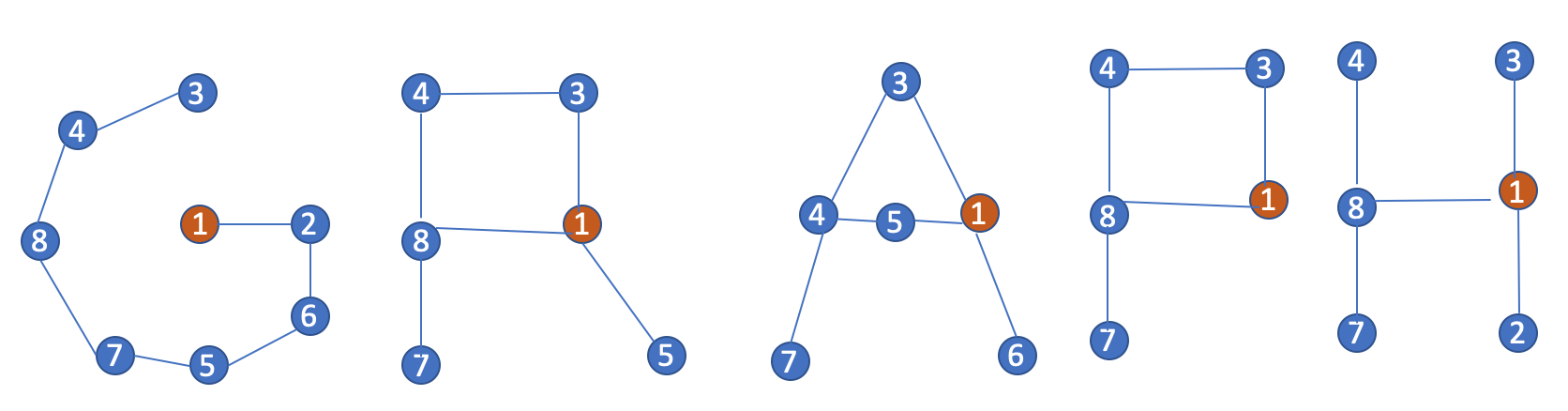Graph Attention NetworkRecently I implemented GAT with the dgl package. For me,knowing the input and output dimensions from the computation helps a lot in understanding the algorithm.

Imagine we have an undirected graph with 7 nodes, some are connected, some are not, each node has 31 features.

The graph attention neural network can be dissected into the following components:

1. Update node embeddings

Get updated node embddings by transferring low dimensional node features to higher dimensions (let’s say the higher dimension is 101) to obtain sufficient expressive power. An activation function is applied on top of the linear transformation to model possible linear/non-linear relationships between nodes.

h(i+1) = activation(np.dot(h(i), W(i))

(7, 101) = ( 7, 31) x (31, 101)

(7, 101) is the shape of updated node embeddings. (31, 101) is the shape of the projection weight matrix.

2. Compute pair-wise un-normalized attention score, by concatenating every node with the rest of nodes.

This is where graph attention network differs from graph convolutional network. Instead of aggregating messages from a node’s one hop neighbors in a constant manner, where the weight from one node to another is inferred based on its number of connections, graph attention network first computes pair-wise node embeddings and then dot product it with a 1D learnable weight vector.

The output of the updated node embeddings after step 1 is of shape (7, 101) –> perform node feature pair-wise concatenation –> each node has 101 features, after pair-wise concatenation, each node pair has 101 * 2 features. There are 7 * 7 node pairs containing node self-attention, (7, 7, 101 * 2 ). Reshape (7, 7, 101 * 2) to (49, 102*2).

eij = activation( np.dot( node_concatenation, learned_weight_vector))

Below shows the input and output dimensions of the above formula:

(49, 101 * 2) * (101 * 2, 1) –> (49, 1) –> (7,7)

where (101 * 2, 1) is the shape of learnable weight vector, (7, 7) is the shape of un-normalized attention matrix.

3.Mask out non-connected nodes and normalize pairwise attention:

Apply a masking matrix (represented by adjacency matrix) to pair-wise attention using element-wise multiplication:

(7, 7) un-normalized attention matrix @ (7, 7) masking matrix = (7, 7) masked attention matrix.

Apply softmax operation on (7, 7) masked attention matrix to getinormalized attention matrix - the attention of node to the rest of its connected nodes should sum up to 1. The output is still of shape (7, 7), we call it normalized attention matrix.

4.Aggregate node information using attention

h(i+2) = activation (np.dot (normalized_attention_matrix, updated_node_embeddings))

(7, 7) * (7, 101) –> (7, 101)

Note that the input node orders have no influence on the above learnable variables - the learnable variables are of shape (31, 101) and (101 * 2, 1). Therefore the node relationships are not learned but rather inferred from the intermedicate steps. Message passing was done in the steps of 3 and 4, where adjacency matrix was first element-wise applied to the un-normalized attention matrix, then normalized, then dot product with the updated node embeddings.

Other resources:

Graph attention networks paper

Dgl tutorials on GAT

People who have given me help on this project: Eric Ma, Cihan Soylu